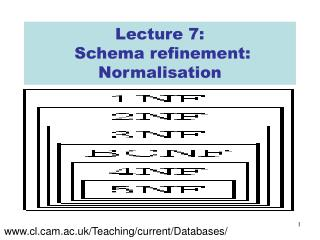DownloadDownload PresentationLecture 7: Schema refinement: Normalisation

Lecture 7: Schema refinement: Normalisation

Télécharger la présentationLecture 7: Schema refinement: Normalisation

- - - - - - - - - - - - - - - - - - - - - - - - - - - E N D - - - - - - - - - - - - - - - - - - - - - - - - - - -
Presentation Transcript

1. Lecture 7: Schema refinement: Normalisation www.cl.cam.ac.uk/Teaching/current/Databases/

2. Decomposing relations • In previous lecture, we saw that we could ‘decompose’ the bad relation schema Data(sid,sname,address,cid,cname,grade) to a ‘better’ set of relation schema Student(sid,sname,address) Course(cid,cname) Enrolled(sid,cid,grade)

3. Are all decompositions good? • Consider our motivating example: Data(sid,sname,address,cid,cname,grade) • Alternatively we could decompose into R1(sid,sname,address) R2(cid,cname,grade) • But this decomposition loses information about the relationship between students and courses

4. Decomposition • A decomposition of a relation R=R(A1:1, …, An:n) is a collection of relations {R1, …, Rk} and a set of queries This is Tim’s somewhat non-standard definition…. such that if then

5. Special Case: Lossless-join decomposition • {R1,…,Rk} is a lossless-joindecomposition of R with respect to an FD set F, if for every relation instance r of R that satisfies F, R1(r) V … V Rk(r) = r (this means project on the attributes of the relation’s schema)

6. Lossless-join: Example 2 • Lossless-join?

7. Lossless-join: Example What happens if we decompose on (sid,sname,address) and (cid,cname,grade)?

8. Dependency preservation • Intuition: If R is decomposed into R1, R2 and R3, say, and we enforce the FDs that hold individually on R1, on R2 and on R3, then all FDs that were given to hold on R must also hold • Reason: Otherwise checking updates for violation of FDs may require computing joins 

9. Dependency preservation • The projection of an FD set F onto a set of attributes Z, written Fz is defined {XY | XYF+ and XYZ} • A decomposition ={R1,…,Rk} is dependency preserving if F+=(FR1 …  FRk)+ GOAL OF SCHEMA REFINEMENT: REDUCE REDUNDANCY WHILE PRESERVING DEPENDENCIES IN A LOSSLESS-JOIN MANNER.

10. Dependency preservation: example • Take R=R(city, street&no, zipcode) with FDs: • city,street&no  zipcode • zipcode  city • Decompose to • R1(street&no,zipcode) • R2(city,zipcode) • Claim: This is a lossless-join decomposition • Is it dependency preserving?

11. Boyce-Codd normal form“Represent Every Fact Only ONCE” • A relation R with FDs F is said to be in Boyce-Codd normal form (BCNF) if for all XA in F+ then • Either AX (‘trivial dependency’), or • X is a superkey for R • Intuition: A relation R is in BCNF if the left side of every non-trivial FD contains a key

12. BCNF: Example • Consider R=R(city, street&no, zipcode) with FDs: • city,street&no  zipcode • zipcode  city • This is not in BCNF, because zipcode is not a superkey for R • We potentially duplicate information relating zipcodes and cities 

13. BCNF: Example BankerSchema(brname,cname,bname) • With FDs • bname  brname • brname,cname  bname • Not in BCNF (Why?) • We might decompose to • BBSchema(bname,brname) • CBrSchema(cname,bname) • This is in BCNF  • BUT this is not dependency-preserving 

14. Third normal form • A relation R with FDs F is said to be in third normal form (3NF) if for all XA in F+ then • Either AX (‘trivial dependency’), or • X is a superkey for R, or • A is a member of some candidate key for R • Notice that 3NF is strictly weaker than BCNF • (A primeattribute is one which appears in a candidate key) • It is always possible to find a dependency-preserving lossless-join decomposition that is in 3NF.

15. 3NF: Example • Recall R=R(city, street&no, zipcode) with FDs: • city,street&no  zipcode • zipcode  city • We saw earlier that this is not in BCNF • However this is in 3NF, because city is a member of a candidate key ({city,street&no})

16. Prehistory: First normal form • First normal form (1NF) is now considered part of the formal definition of the relational model • It states that the domain of all attributes must be atomic (indivisible), and that the value of any attribute in a tuple must be a single value from the domain • NOTE: Modern databases have moved away from this restriction

17. Prehistory: Second normal form • A partial functional dependency XY is an FD where for some attribute AX, (X-{A})Y • A relation schema R is in second normal form(2NF) if every non-prime attribute A in R is not partially dependent on any key of R

18. Summary: Normal forms 1NF 2NF 3NF BCNF

19. Not the end of problems… • ONLY TRIVIAL FDs!! (see Date) • Is in BCNF! • Obvious insertion anomalies…

20. Decomposition • Even though its in BCNF, we’d prefer to decompose it to the schema • Teaches(Course,Teacher) • Books(Course,Title) • We need to extend our underlying theory to capture this form of redundancy

21. Further normal forms • We can generalise the notion of FD to a ‘multi-valued dependency’, and define two further normal forms (4NF and 5NF) • These are detailed in the textbooks • In practise, BCNF (preferably) and 3NF (at the very least) are good enough

22. Design goals: Summary • Our goal for relational database design is • BCNF • Lossless-join decomposition • Dependency preservation • If we can’t achieve this, we accept • Lack of dependency preservation, or • 3NF

23. Summary You should now understand: • Decomposition of relations • Lossless-join decompositions • Dependency preserving decompositions • BCNF and 3NF • 2NF and 1NF Next lecture: More algebra, more SQL